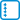## Rectangle tiling

How many ways can you tile a rectangle $$n \times k$$ by using tiles $$1 \times 2$$?

• #### Variant

Solve for $$k=2$$.

• #### Variant

And how many different ways of tiling by the same tiles has the rectangle when $$k=3$$?